# Load Flow and Y Bus

### Formation of Bus Admittance Matrix (Ybus)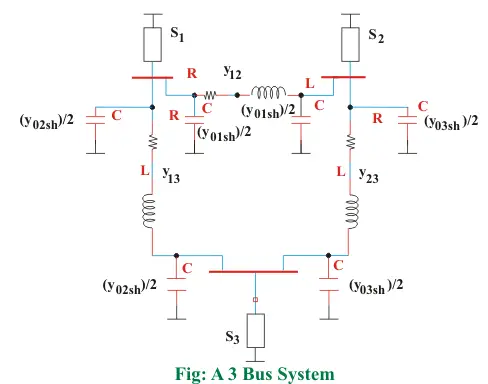S1, S2, S3 are net complex power injections into bus 1, 2, 3 respectively
y12, y23, y13 are line admittances between lines 1-2, 2-3, 1-3
y01sh/2, y02sh/2, y03sh/2 are half-line charging admittance between lines 1-2, 1-3 and 2-3

The half-line charging admittances connected to the same bus are at same potential and thus can be combined into one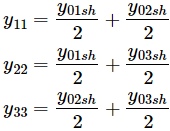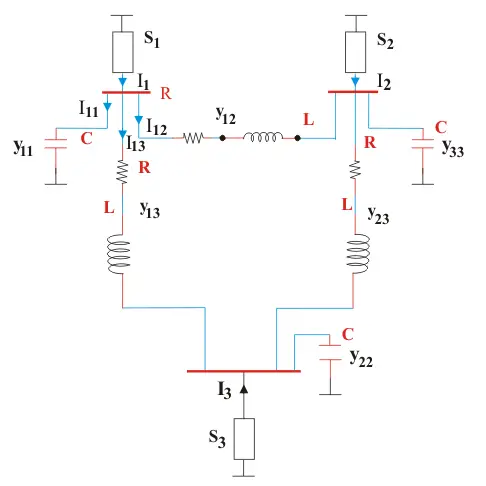If we apply KCL at bus 1, we have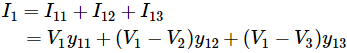Where, V1, V2, V3 are voltage values at bus 1, 2, 3 respectively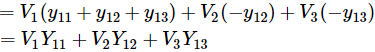Where,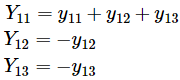Similarly by applying KCL at buses 2 and 3 we can derive the values of I2 and I3
Finally we have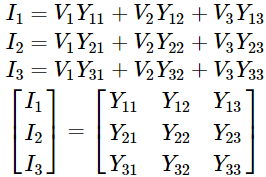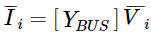In general for an n bus system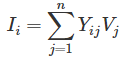Some observations on YBUS matrix:

1. YBUS is a sparse matrix
2. Diagonal elements are dominating
3. Off diagonal elements are symmetric
4. The diagonal element of each node is the sum of the admittances connected to it
5. The off diagonal element is negated admittance

### Development of Load Flow Equations

The net complex power injection at bus i is given by: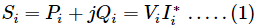Taking conjugate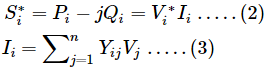Substituting the value of Ii in equation (2)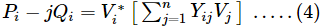To derive the static load flow equation in polar form in equation (4) substitute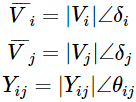On substitution of the above values equation (4) becomes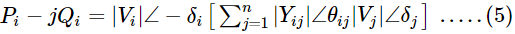In equation (5) on multiplication of the terms angles get added. Let’s denote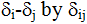for convenience
Therefore equation (5) becomes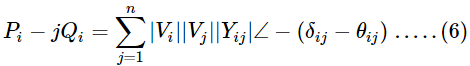Expansion of equation (6) into sine and cosine terms gives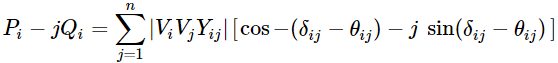Equating real and imaginary parts we get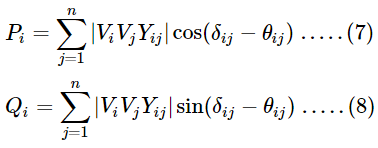Equations (7) and (8) are the static load flow equations in polar form. The above obtained equations are non-linear algebraic equations and can be solved using iterative numerical algorithms.
Similarly to obtain load flow equations in rectangular form in equation (4) substitute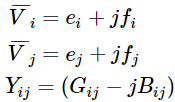On substituting above values into equation (4) and equating real and imaginary parts we get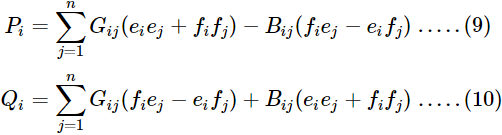Equations (9) and (10) are static load flow equations in rectangular form.

Want To Learn Faster? 🎓
Get electrical articles delivered to your inbox every week.
No credit card required—it’s 100% free.